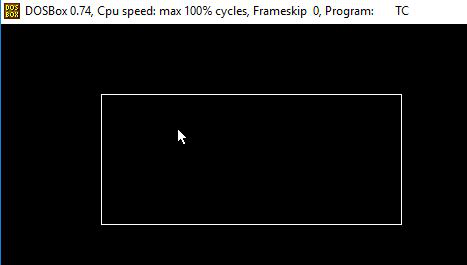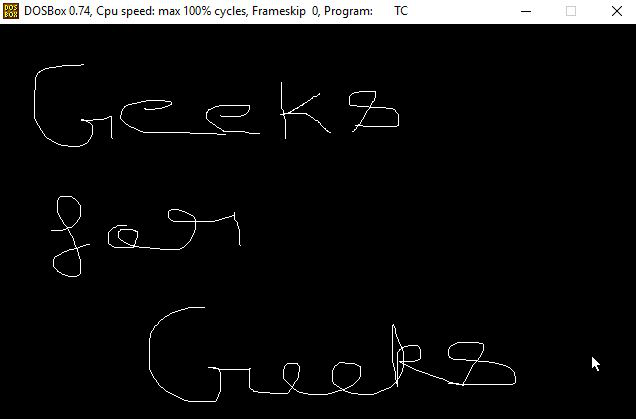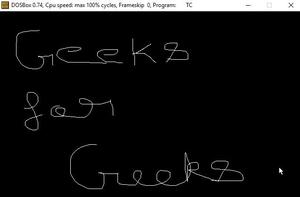GFG App
Open AppBrowser
Continue

# Use cases of mouse programming in C/C++ | Set 2

In this article, we will discuss some use cases of Mouse Programming:

### Restrict mouse pointer:

The mouse pointer can be restricted in particular rectangle. The idea is to create a function called restrictmouse() which takes four parameters which containing X coordinate and Y coordinate. First point mention the top of the rectangle and the second point mention the bottom of the rectangle. Below are the functions used for the same:

• initmouse(): use to initialize mouse.
• showmouse(): shows the mouse pointer on the output screen.
• restrictmouse(): used to set Horizontal and vertical limit of the mouse pointer by setting the following parameters. AX = 7 for horizontal and AX = 8 for vertical.

Below is the program for the same:

## C

 `// C program to restrict the mouse ` `// pointer ` `#include ` `#include ` `#include ` `#include ` `union` `REGS in, out; ` ` `  `// Function to initialize the mouse ` `// pointer using graphics ` `int` `initMouse() ` `{ ` `    ``in.x.ax = 0; ` `    ``int86(0X33, &in, &out); ` ` `  `    ``return` `out.x.ax; ` `} ` ` `  `// Function to display the mouse ` `// pointer using graphics ` `void` `showMouse() ` `{ ` `    ``in.x.ax = 1; ` `    ``int86(0X33, &in, &out); ` `} ` ` `  `// Function to restrict the mouse ` `// pointers ` `void` `restrictMouse(``int` `x1, ``int` `y1, ` `                   ``int` `x2, ``int` `y2) ` `{ ` `    ``// Set Horizontal limit ` `    ``in.x.ax = 7; ` `    ``in.x.cx = x1; ` `    ``in.x.dx = x2; ` `    ``int86(0X33, &in, &out); ` ` `  `    ``// Set Vertical limit ` `    ``in.x.ax = 8; ` `    ``in.x.cx = y1; ` `    ``in.x.dx = y2; ` `    ``int86(0X33, &in, &out); ` `} ` ` `  `// Driver Code ` `void` `main() ` `{ ` `    ``int` `status, i, gd = DETECT, gm; ` ` `  `    ``// Initialize graphics ` `    ``initgraph(&gd, &gm, ``"C:\\TURBOC3\\BGI"``); ` ` `  `    ``// Get the status of the mouse ` `    ``status = initMouse(); ` ` `  `    ``// Check if mouse is available or not ` `    ``if` `(status == 0) ` `        ``printf``(``"Mouse support "` `               ``"not available.\n"``); ` `    ``else` `{ ` `        ``showMouse(); ` ` `  `        ``// Draw rectangle for displaying ` `        ``// the boundary ` `        ``rectangle(100, 70, 400, 200); ` `        ``restrictMouse(100, 70, 400, 200); ` `    ``} ` `    ``getch(); ` ` `  `    ``// Close the graphics ` `    ``closegraph(); ` ` `  `    ``return` `0; ` `}`

## C++

 `// C++ program to restrict the mouse ` `// pointer ` `#include ` `#include ` `#include ` `#include ` `#include ` `using` `namespace` `std; ` ` `  `union` `REGS in, out; ` ` `  `// Function to initialize the mouse ` `// pointer using graphics ` `int` `initMouse() ` `{ ` `    ``in.x.ax = 0; ` `    ``int86(0X33, &in, &out); ` ` `  `    ``return` `out.x.ax; ` `} ` ` `  `// Function to display the mouse ` `// pointer using graphics ` `void` `showMouse() ` `{ ` `    ``in.x.ax = 1; ` `    ``int86(0X33, &in, &out); ` `} ` ` `  `// Function to restrict the mouse ` `// pointers ` `void` `restrictMouse(``int` `x1, ``int` `y1, ` `                   ``int` `x2, ``int` `y2) ` `{ ` `    ``// Set Horizontal limit ` `    ``in.x.ax = 7; ` `    ``in.x.cx = x1; ` `    ``in.x.dx = x2; ` `    ``int86(0X33, &in, &out); ` ` `  `    ``// Set Vertical limit ` `    ``in.x.ax = 8; ` `    ``in.x.cx = y1; ` `    ``in.x.dx = y2; ` `    ``int86(0X33, &in, &out); ` `} ` ` `  `// Driver Code ` `void` `main() ` `{ ` `    ``int` `status, i, gd = DETECT, gm; ` ` `  `    ``// Initialize graphics ` `    ``initgraph(&gd, &gm, ``"C:\\TURBOC3\\BGI"``); ` ` `  `    ``// Get the status of the mouse ` `    ``status = initMouse(); ` ` `  `    ``// Check if mouse is available or not ` `    ``if` `(status == 0) ` `        ``cout << ``"Mouse support "` `             ``<< ``"not available.\n"``; ` `    ``else` `{ ` `        ``showMouse(); ` ` `  `        ``// Draw rectangle for displaying ` `        ``// the boundary ` `        ``rectangle(100, 70, 400, 200); ` `        ``restrictMouse(100, 70, 400, 200); ` `    ``} ` `    ``getch(); ` ` `  `    ``// Close the graphics ` `    ``closegraph(); ` ` `  `    ``return` `0; ` `}`

Output:### Free hand drawing:

This following program makes use of some sub function, which were already discussed previously, and shows how they can be used to write useful programs like free-hand drawing. Below are the functions used:

• initmouse(): use to initialize mouse.
• showmouse(): shows mouse pointer on the output screen.
• hidemouse(): used to hide mouse while drawing.
• getmouseposition(): Fetches current location of the pointer and draw line accordingly.

Below is the program for the same:

## C

 `// C program to perform Free Hand Drawing ` `// using mouse programming ` `#include ` `#include ` `#include ` `union` `REGS in, out; ` ` `  `// Function to initialize the mouse ` `// pointer using graphics ` `int` `initMouse() ` `{ ` `    ``in.x.ax = 0; ` `    ``int86(0X33, &in, &out); ` ` `  `    ``return` `out.x.ax; ` `} ` ` `  `// Function to display the mouse ` `// pointer using graphics ` `void` `showMouse() ` `{ ` `    ``in.x.ax = 1; ` `    ``int86(0X33, &in, &out); ` `} ` ` `  `// Function to hide the mouse ` `// pointer using graphics ` `void` `hideMouse() ` `{ ` `    ``// Set AX=2 to hide mouse ` `    ``in.x.ax = 2; ` `    ``int86(0X33, &in, &out); ` `} ` ` `  `// Function to get the exact position ` `// of the mouse ` `getMousePosition(``int``* x, ``int``* y, ` `                 ``int``* click) ` `{ ` `    ``in.x.ax = 3; ` ` `  `    ``// Get the coordinates ` `    ``int86(0x33, &in, &out); ` ` `  `    ``// Update the coordinates ` `    ``*x = out.x.cx; ` `    ``*y = out.x.dx; ` `    ``*click = out.x.bx & 1; ` `} ` ` `  `// Driver Code ` `void` `main() ` `{ ` `    ``int` `status, i, gd = DETECT, gm,x1,y1,x2,y2; ` ` `  `    ``// Initialize graphics ` `    ``initgraph(&gd, &gm, ``"C:\\TURBOC3\\BGI"``); ` ` `  `    ``initMouse(); ` ` `  `    ``// kbhit If a key has been pressed ` `    ``// then it returns a non zero value ` `    ``// otherwise returns zero(false) ` `    ``while` `(!kbhit()) { ` ` `  `        ``// Show the mouse pointer ` `        ``showMouse(); ` ` `  `        ``// Get the mouse position ` `        ``getMousePosition(&x1, &y1, &click); ` `        ``x2 = x1; ` `        ``y2 = y1; ` ` `  `        ``// When mouse is clicked ` `        ``while` `(click == 1) { ` `            ``hideMouse(); ` ` `  `            ``// Draw line ` `            ``line(x1, y1, x2, y2); ` `            ``x1 = x2; ` `            ``y1 = y2; ` ` `  `            ``// Get the mouse position ` `            ``getMousePosition(&x2, &y2, &click); ` `        ``} ` `    ``} ` ` `  `    ``getch(); ` ` `  `    ``// Close the graphics ` `    ``closegraph(); ` ` `  `    ``return` `0; ` `}`

## C++

 `// C++ program to perform Free Hand ` `// Drawing using mouse programming ` `#include ` `#include ` `#include ` `#include ` `using` `namespace` `std; ` ` `  `union` `REGS in, out; ` ` `  `// Function to initialize the mouse ` `// pointer using graphics ` `int` `initMouse() ` `{ ` `    ``in.x.ax = 0; ` `    ``int86(0X33, &in, &out); ` ` `  `    ``return` `out.x.ax; ` `} ` ` `  `// Function to display the mouse ` `// pointer using graphics ` `void` `showMouse() ` `{ ` `    ``in.x.ax = 1; ` `    ``int86(0X33, &in, &out); ` `} ` ` `  `// Function to hide the mouse ` `// pointer using graphics ` `void` `hideMouse() ` `{ ` `    ``// Set AX=2 to hide mouse ` `    ``in.x.ax = 2; ` `    ``int86(0X33, &in, &out); ` `} ` ` `  `// Function to get the exact position ` `// of the mouse ` `getMousePosition(``int``* x, ``int``* y, ` `                 ``int``* click) ` `{ ` `    ``in.x.ax = 3; ` ` `  `    ``// Get the coordinates ` `    ``int86(0x33, &in, &out); ` ` `  `    ``// Update the coordinates ` `    ``*x = out.x.cx; ` `    ``*y = out.x.dx; ` `    ``*click = out.x.bx & 1; ` `} ` ` `  `// Driver Code ` `void` `main() ` `{ ` `    ``int` `status, i, gd = DETECT, gm; ` ` `  `    ``// Initialize graphics ` `    ``initgraph(&gd, &gm, ``"C:\\TURBOC3\\BGI"``); ` ` `  `    ``initMouse(); ` ` `  `    ``// kbhit If a key has been pressed ` `    ``// then it returns a non zero value ` `    ``// otherwise returns zero(false) ` `    ``while` `(!kbhit()) { ` ` `  `        ``// Show the mouse pointer ` `        ``showMouse(); ` ` `  `        ``// Get the mouse position ` `        ``getMousePosition(&x1, &y1, &click); ` `        ``x2 = x1; ` `        ``y2 = y1; ` ` `  `        ``// When mouse is clicked ` `        ``while` `(click == 1) { ` `            ``hideMouse(); ` ` `  `            ``// Draw line ` `            ``line(x1, y1, x2, y2); ` `            ``x1 = x2; ` `            ``y1 = y2; ` ` `  `            ``// Get the mouse position ` `            ``getMousePosition(&x2, &y2, &click); ` `        ``} ` `    ``} ` ` `  `    ``getch(); ` ` `  `    ``// Close the graphics ` `    ``closegraph(); ` ` `  `    ``return` `0; ` `}`

Output:My Personal Notes arrow_drop_up